# ML Aggarwal Class 6 Solutions for ICSE Maths Chapter 4 Playing with Numbers Ex 4.1

## ML Aggarwal Class 6 Solutions for ICSE Maths Chapter 4 Playing with Numbers Ex 4.1

Question 1.
Fill in the blanks:
(i) A number having exactly two factors is called a …..
(ii) A number having more than two factors is called a ………
(iii) 1 is neither ……… nor ………
(iv) The smallest prime number is ………
(v) The smallest odd prime number is ………
(vi) The smallest composite number is ………
(vii) The smallest odd composite number is ………
(viii) All prime numbers (except 2) are ………
Solution:Question 2.
State whether the following statements are ture (T) or false (F):
(i) The sum of three odd numbers is an even number.
(ii) The sum of two odd numbers and one even number is an even number.
(iii) The product of two even numbers is always an even number.
(iv) The product of three odd numbers is an odd number.
(v) If an even number is divided by 2, the quotient is always an odd number.
(vi) All prime numbers are odd.
(vii) All even numbers are composite.
(viii) Prime numbers do not have any factors.
(ix) A natural number is called a composite number if it has atleast one more factor other than 1 and the number itself.
(x) Two consecutive numbers cannot be both prime.
(xi) Two prime numbers are always co-prime numbers.
Solution: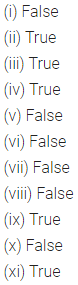Question 3.
Write all the factors of the following natural numbers:
(i) 68
(ii) 27
(iii) 210
Solution:Question 4.
Write first six multiples of the following natural numbers:
(i) 3
(ii) 5
(iii) 12
Solution: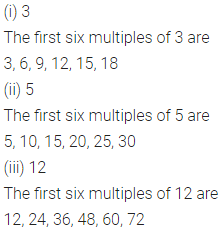Question 5.
Match the items in column 1 with the items in column 2:Solution: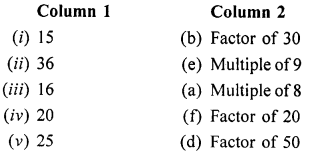Question 6.
Find the common factors of :
(i) 20 and 28
(ii) 35 and 50
(iii) 56 and 120
Solution: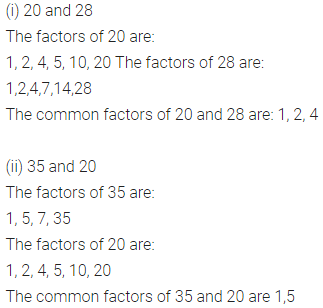Question 7.
Find the common factors of:
(i) 4, 8, 12
(ii) 10, 30 and 45
Solution: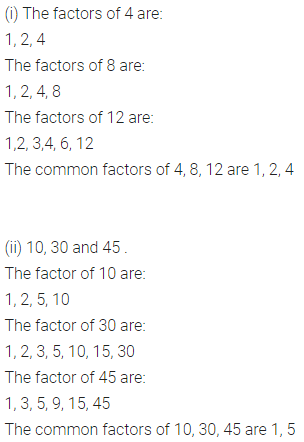Question 8.
Write all natural numbers less than 100 which are common
multiples of 3 and 4.
Solution:Question 9.
(i) Write the odd numbers between 36 and 53.
(ii) Write the even numbers between 232 and 251.
Solution:Question 10.
(i) Write four consecutive odd numbers succeeding 79.
(ii) Write three consecutive even numbers preceding 124.
Solution: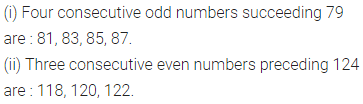Question 11.
What is greatest prime number between 1 and 15?
Solution:Question 12.
Which of the following numbers are prime?
(i) 29
(ii) 57
(iii) 43
(iv) 61
Solution: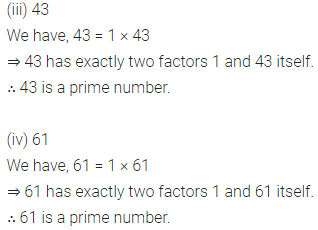Question 13.
Which of the following pairs of numbers are co-prime?
(i) 12 and 35
(ii) 15 and 37
(iii) 27 and 32
(iv) 17 and 85
(v) 515 and 516
(vi) 215 and 415
Solution: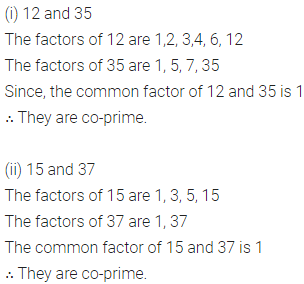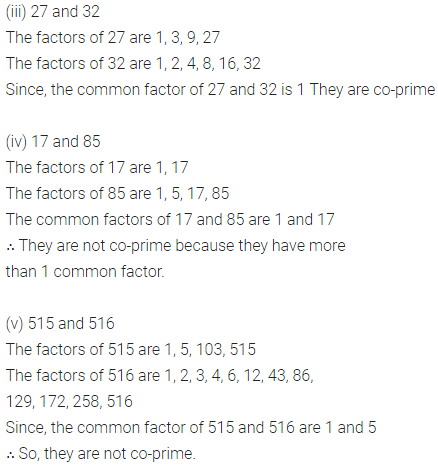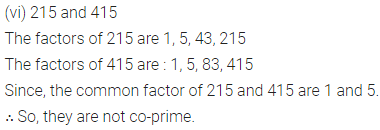Question 14.
Express each of the following numbers as the sum of two odd primes:
(i) 24
(ii) 36
(iii) 84
(iv) 98
Solution: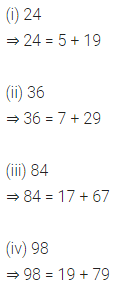Question 15.
Express each of the following numbers as the sum of twin-primes:
(i) 24
(ii) 36
(iii) 84
(iv) 120
Solution: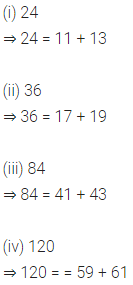Question 16.
Express each of the following numbers as the sum of three odd primes:
(i) 21
(ii) 35
(iii) 49
(iv) 63
Solution: